Working with DataFrames and code examples from the practice session¶

In :
import pandas as pd
import numpy as np
import matplotlib.pyplot as plt
from IPython.display import display # Print like jupyter notebook's default cell output using display()
In :
# A toy dataset with two attributes.
df = pd.DataFrame(
{'group':['group_1','group_2','group_3','group_4','group_5','group_6'],
'count':[7,9,4,3,8,20],
})
df
Out:
group count
0 group_1 7
1 group_2 9
2 group_3 4
3 group_4 3
4 group_5 8
5 group_6 20

Selecting a row (an instance) from the dataset¶

We can select an instance by index using pandas.DataFrame iloc. Index values start from 0

In :
#Therefore, in order to select an instance from row 1 you have to select the instance from index 0
df.iloc
Out:
group    group_1
count          7
Name: 0, dtype: object

You can use a list to get multiple rows by index

In :
# Row with index=2 and index=0
df.iloc[[2, 0]]
Out:
group count
2 group_3 4
0 group_1 7

Or you can split the data by index

In :
# Select instances (rows) from index 1 to the end
df.iloc[1:]
Out:
group count
1 group_2 9
2 group_3 4
3 group_4 3
4 group_5 8
5 group_6 20
In :
# Select instances (rows) from index 1 to the end excluding last (-1)
df.iloc[1:-1]
Out:
group count
1 group_2 9
2 group_3 4
3 group_4 3
4 group_5 8

Select all rows and all columns, except last column.

In :
df.iloc[:,:-1]
Out:
group
0 group_1
1 group_2
2 group_3
3 group_4
4 group_5
5 group_6

Select all rows and all columns, except last two columns.

In :
df.iloc[:,:-2]
Out:
0
1
2
3
4
5

iloc will allow you to get rows using a boolean vector

In :
# Same size as the dataset
df.iloc[[True,False,False,False,True,True]]
Out:
group count
0 group_1 7
4 group_5 8
5 group_6 20

Similar results can be achieved with pandas.DataFrame.loc

In :
print(df.loc)
display(df.loc[[2, 0]])
display(df.loc[[True,False,False,False,True,True]])
group    group_1
count          7
Name: 0, dtype: object
group count
2 group_3 4
0 group_1 7
group count
0 group_1 7
4 group_5 8
5 group_6 20

You can generate a boolean vector using a comparison operator

In :
print(df['count'] > 3)
0     True
1     True
2     True
3    False
4     True
5     True
Name: count, dtype: bool

Only loc will allow you to combinig previous two directly. pandas.DataFrame.loc is primarily label based, but may also be used with a boolean array. (For iloc you should call values).

In :
display(df.loc[df['count'] > 3])
display(df.iloc[(df['count'] > 3).values])
group count
0 group_1 7
1 group_2 9
2 group_3 4
4 group_5 8
5 group_6 20
group count
0 group_1 7
1 group_2 9
2 group_3 4
4 group_5 8
5 group_6 20

We can also use logical operators

In :
df.loc[(df['count'] > 3) & (df['count'] < 9)]
Out:
group count
0 group_1 7
2 group_3 4
4 group_5 8

Select only some of the columns

In :
# df.loc[condition, columns]
df.loc[(df['count'] > 3) & (df['count'] < 9), ['count']]
Out:
count
0 7
2 4
4 8

If you would like to change the dataFrame. E.g. remove instances from the dataset (e.g. outliers).

In :
df = df[df['count'] < 10]
df
Out:
group count
0 group_1 7
1 group_2 9
2 group_3 4
3 group_4 3
4 group_5 8

Major diffetence, loc will allow you to access a group of rows and columns by label(s).

In :
# Let's set the column 'group' as a index
df2 = df.set_index('group')

# Select rows by group column
df2.loc[['group_1','group_2']]
Out:
count
group
group_1 7
group_2 9
In :
# Will give an error invalid literal for int() with base 10: 'group_1'
# df2.iloc[['group_1','group_2']]

The iloc still allows you to select a row by an index.

In :
df2.iloc
Out:
count    9
Name: group_2, dtype: int64

Main difference - iloc selects by position (integer-location based indexing), loc selects by label (column index)

In :
df = df.sort_values(by='count', ascending=False)
display(df)
display(df.iloc)
display(df.loc)
group count
1 group_2 9
4 group_5 8
0 group_1 7
2 group_3 4
3 group_4 3
group    group_2
count          9
Name: 1, dtype: object
group    group_1
count          7
Name: 0, dtype: object

In :
# We can specify a delimiter to use. Attribute values in a file may be separated by a comma, by a tab or something else.
# In the Titanic dataset attribute values are separated by a comma

# delimiter is an alternative argument name for sep.

# In the adult dataset attribute values are separated by a tab

Group by¶

You can use a pandas group by function to get a very good overview of the data. Let's see how many people were in different classes

In :
# We group by occupation and count the instances in each class
# agg = aggregate using one or more operations over the specified axis
Out:
count
occupation
? 1843
Armed-Forces 9
Craft-repair 4099
Exec-managerial 4066
Farming-fishing 994
Handlers-cleaners 1370
Machine-op-inspct 2002
Other-service 3295
Priv-house-serv 149
Prof-specialty 4140
Protective-serv 649
Sales 3650
Tech-support 928
Transport-moving 1597

The previous results can be achieved also with count(). However, this will not return a dataframe.

In :
Out:
occupation
?                    1843
Armed-Forces            9
Craft-repair         4099
Exec-managerial      4066
Farming-fishing       994
Handlers-cleaners    1370
Machine-op-inspct    2002
Other-service        3295
Priv-house-serv       149
Prof-specialty       4140
Protective-serv       649
Sales                3650
Tech-support          928
Transport-moving     1597
Name: occupation, dtype: int64

The previous results can be achieved also with value_counts(). However, this will not return a dataframe.

In :
Out:
Prof-specialty       4140
Craft-repair         4099
Exec-managerial      4066
Sales                3650
Other-service        3295
Machine-op-inspct    2002
?                    1843
Transport-moving     1597
Handlers-cleaners    1370
Farming-fishing       994
Tech-support          928
Protective-serv       649
Priv-house-serv       149
Armed-Forces            9
Name: occupation, dtype: int64

Groupby and agg allows us to do more complicated analysis. E.g., you can use aggregate with more than one operation

In :
adult_df.groupby(['occupation'])['salaries'].agg(['count', 'mean' ,'median', 'max', np.nanmean, np.sum])
Out:
count mean median max nanmean sum
occupation
? 1843 34003.842742 31552.0 81615.0 34003.842742 6.266908e+07
Adm-clerical 3770 35128.035036 31662.0 140000.0 35128.035036 1.324327e+08
Armed-Forces 9 34740.000000 31983.0 67993.0 34740.000000 3.126600e+05
Craft-repair 4099 39317.777186 34461.0 90774.0 39317.777186 1.611636e+08
Exec-managerial 4066 47995.081407 44789.0 86832.0 47995.081407 1.951480e+08
Farming-fishing 994 34930.842768 32299.5 81443.0 34930.842768 3.472126e+07
Handlers-cleaners 1370 32607.360011 30947.0 88601.0 32607.360011 4.467208e+07
Machine-op-inspct 2002 35230.203830 32033.5 100000.0 35230.203830 7.053087e+07
Other-service 3295 31997.697257 30915.0 86434.0 31997.697257 1.054324e+08
Priv-house-serv 149 30275.214765 30848.0 63525.0 30275.214765 4.511007e+06
Prof-specialty 4140 46747.103623 41331.0 89686.0 46747.103623 1.935330e+08
Protective-serv 649 42567.922958 36038.0 91182.0 42567.922958 2.762658e+07
Sales 3650 40455.787945 34542.0 88861.0 40455.787945 1.476636e+08
Tech-support 928 41839.278017 35138.5 83933.0 41839.278017 3.882685e+07
Transport-moving 1597 37954.787454 33190.0 90737.0 37954.787454 6.061380e+07

You can use aggregate to plot two different attributes to a single plot

In :
from matplotlib import rcParams
rcParams['figure.figsize'] = 5, 5

gender.plot(kind="bar")
plt.show()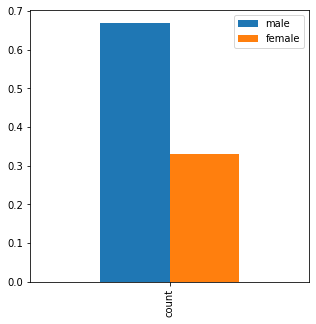We could also group by all the columns. First, we group by class then by age then by gender and at last by survived.

In :
titanic_df.groupby(['Class','Age','Sex','Survived'])['Survived'].agg(['count'])
Out:
count
Class Age Sex Survived
Yes 140
Male No 118
Yes 57
Child Female Yes 1
Male Yes 5
Yes 80
Male No 154
Yes 14
Child Female Yes 13
Male Yes 11
Yes 76
Male No 387
Yes 75
Child Female No 17
Yes 14
Male No 35
Yes 13
Yes 20
Male No 670
Yes 192

If we would like to get relative frequency, we just have to divide by the number of rows in the data

In :
grouped = titanic_df.groupby(['Class','Age','Sex','Survived'])['Survived'].agg(['count']) / len(titanic_df)
print('total ', grouped['count'].sum())
grouped
total  1.0
Out:
count
Class Age Sex Survived
Yes 0.063607
Male No 0.053612
Yes 0.025897
Child Female Yes 0.000454
Male Yes 0.002272
Yes 0.036347
Male No 0.069968
Yes 0.006361
Child Female Yes 0.005906
Male Yes 0.004998
Yes 0.034530
Male No 0.175829
Yes 0.034075
Child Female No 0.007724
Yes 0.006361
Male No 0.015902
Yes 0.005906
Yes 0.009087
Male No 0.304407
Yes 0.087233
To get information which methods are available to an object press tab after the dot/period. Also, by pressing shift+tab after the function, it will give you information.
In :
from IPython.display import Image
In :
Image(filename='tab.jpg')
Out: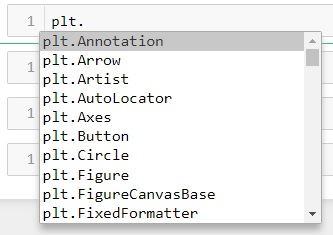In :
Image(filename='description.jpg')
Out: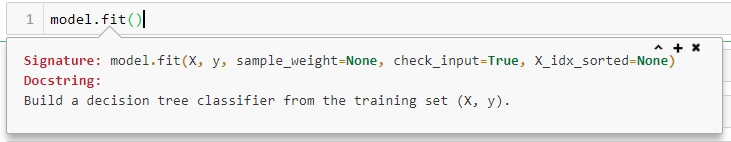Cleaning the data¶

Sometimes values include leading and/or trailing spaces. Remove leading and trailing whitespace from strings. We could use the strip(). The method strip() returns a copy of the string in which by default whitespace characters have been stripped from the beginning and the end of the string. However, the method strip() but it can only be used on strings. Therefore, first, we have to select only columns that have string values.

In :
df_obj = adult_df.select_dtypes(['object'])  # Get columns with objects aka strings

# Let's select only columns containing string values and apply a function. Lambdas are anonymous one-line functions.

In order to replace non-sensical values or typos, we can use replace method to replace values.

In :

Change data type¶

Sometimes we would like to change for example string values into categories and order them according to our domain knowledge

In :
type = pd.api.types.CategoricalDtype(ordered=True, categories = ["1st", "2nd", "Crew" , "3rd"])
titanic_df['Class'] = titanic_df['Class'].astype(type)
titanic_df['Class'].dtype
Out:
CategoricalDtype(categories=['1st', '2nd', 'Crew', '3rd'], ordered=True)

Objects to DataFrame¶

Sometimes algorithms may output some quite complicated objects (in our case generator object) and it is often a good idea to put the object into a dataframe for several reasons (e.g. sorting).

In :
from orangecontrib.associate import *
from apyori import apriori

titanic = titanic_df.values # Convert dataFrame into Array
generator_object = apriori(titanic, min_support = 0.000001, min_confidence = 0.000001) # run apriori

df_data = []
for record in list(generator_object):
support = record
rules = record

for rule in rules:
rule = list(rule)
rule.append(support)
df_data.append(rule)

df_apriori = pd.DataFrame(df_data)
df_apriori.columns = ["items_base (antecedent)","items_add (consequent)", "confidence", "lift", "support"]
Out:
items_base (antecedent) items_add (consequent) confidence lift support
276 (2nd, Yes, Male) (Child) 0.440000 8.884771 0.004998
132 (2nd, Yes) (Child) 0.203390 4.106982 0.010904
259 (Yes, 2nd, Adult) (Female) 0.851064 3.985514 0.036347
138 (2nd, Yes) (Female) 0.788136 3.690822 0.042254
273 (Child, Yes, Female) (2nd) 0.464286 3.585589 0.005906
235 (Yes, 1st, Adult) (Female) 0.710660 3.328005 0.063607
105 (1st, Yes) (Female) 0.694581 3.252709 0.064062
133 (Child, Yes) (2nd) 0.421053 3.251708 0.010904
296 (No, 3rd, Female) (Child) 0.160377 3.238446 0.007724
159 (3rd, Female) (Child) 0.158163 3.193737 0.014085

Machine Learning¶

In :

K-means¶

In :
from sklearn.cluster import KMeans
cluster_assignments = KMeans(n_clusters=10, random_state=1).fit_predict(digits.data)

Use heatmap to visualize the results

In :
import seaborn as sns
from matplotlib import rcParams

rcParams['figure.figsize'] = 16, 10
tab = pd.crosstab(cluster_assignments,  digits.target)
sns.heatmap(tab, annot=True, annot_kws={'size':20}, fmt='g')
plt.xlabel('target / real value')
plt.ylabel('cluster number')
plt.show()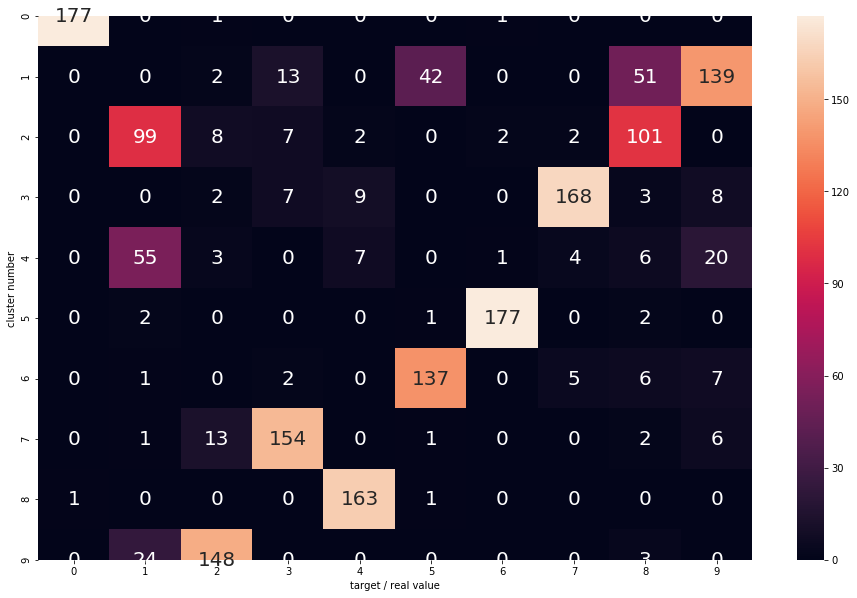Split data into training and test dataset¶

In :
from sklearn.model_selection import train_test_split

# As splitting is random, we use random_state=0 to get same splits each time
# You have to specify only train_size or test_size or you can specify both.
X_train, X_test, y_train, y_test = train_test_split(digits.data, digits.target, train_size=0.7, test_size=0.3, random_state=0)

KNN¶

In :
from sklearn.neighbors import KNeighborsClassifier
model = KNeighborsClassifier(n_neighbors = 1)

# Train (fit) a model
model.fit(X_train, y_train)

# Predict label for the 2nd instance from the test set
model.predict([X_test])
Out:
array()

Decision tree¶

In :
from sklearn.tree import DecisionTreeClassifier
model = DecisionTreeClassifier(random_state=0, max_depth=4, criterion='gini')

# Train (fit) a model
model.fit(X_train, y_train)

# Predict label for the 2nd instance from the test set
model.predict([X_test])

# Get probabilities for the 2nd instance from the test set
prob = model.predict_proba([X_test])
prob
Out:
array([[0.        , 0.21400778, 0.43968872, 0.05058366, 0.0155642 ,
0.01167315, 0.0311284 , 0.04280156, 0.18677043, 0.0077821 ]])

Evaluate classifier¶

TP, FN, FP, TN and calculate accuracy, precision, recall¶

In :
Out:
ID A B Actual
0 1 0 1 1
1 2 0 0 1
2 3 1 0 0
3 4 0 1 1
4 5 1 1 1

Solution 1 - with groupby

In :
TP = data.loc[data['Actual']==1].groupby('A')['A'].count()
FN = data.loc[data['Actual']==1].groupby('A')['A'].count()
FP = data.loc[data['Actual']==0].groupby('A')['A'].count()
TN = data.loc[data['Actual']==0].groupby('A')['A'].count()

accuracy = (TN + TP)/(TP + TN + FP + FN)
precision = TP / (TP + FP)
recall = TP / (TP + FN)

print("A Accuracy: ", accuracy)
print("A Precision: ", precision)
print("A Recall: ", recall)
A Accuracy:  0.515
A Precision:  0.5112781954887218
A Recall:  0.68

Solution 2 - crosstab

In :
crossA = pd.crosstab(data['Actual'], data['A'], colnames=['A'], rownames=['Actual'], margins=True)
TP = crossA.loc[1,1]
TN = crossA.loc[0,0]
FN = crossA.loc[1,0]
FP = crossA.loc[0,1]

accuracy = (TN + TP)/(TP + TN + FP + FN)
precision = TP / (TP + FP)
recall = TP / (TP + FN)

print("A Accuracy: ", accuracy)
print("A Precision: ", precision)
print("A Recall: ", recall)

crossA
A Accuracy:  0.515
A Precision:  0.5112781954887218
A Recall:  0.68
Out:
A 0 1 All
Actual
0 35 65 100
1 32 68 100
All 67 133 200

Solution 3 - sklearn.confusion_matrix()

In :
from sklearn.metrics import confusion_matrix

confusion_matrix_result = confusion_matrix(data['Actual'], data['A'], labels=[1,0]) # labels=[1,0] display matrix as we are used to
print(confusion_matrix_result)

TN, FP, FN, TP = confusion_matrix_result.ravel()

accuracy = (TN + TP)/(TP + TN + FP + FN)
precision = TP / (TP + FP)
recall = TP / (TP + FN)

print("A Accuracy: ", accuracy)
print("A Precision: ", precision)
print("A Recall: ", recall)
[[68 32]
[65 35]]
A Accuracy:  0.515
A Precision:  0.5223880597014925
A Recall:  0.35

Solution 4 - sklearn.metrics

In :
from sklearn.metrics import accuracy_score
from sklearn.metrics import precision_score
from sklearn.metrics import recall_score

acc = accuracy_score(data['Actual'], data['A'])
print('acc =',acc)

prec = precision_score(data['Actual'], data['A'])
print('precision =',prec)

recall = recall_score(data['Actual'], data['A'])
print('recall =',recall)
acc = 0.515
precision = 0.5112781954887218
recall = 0.68

ROC Curve¶

In :
from sklearn.utils import shuffle
# Train and validation dataset
sns.scatterplot('V1', 'V2', data=shuffle(train), hue='y').axis('equal')
plt.show()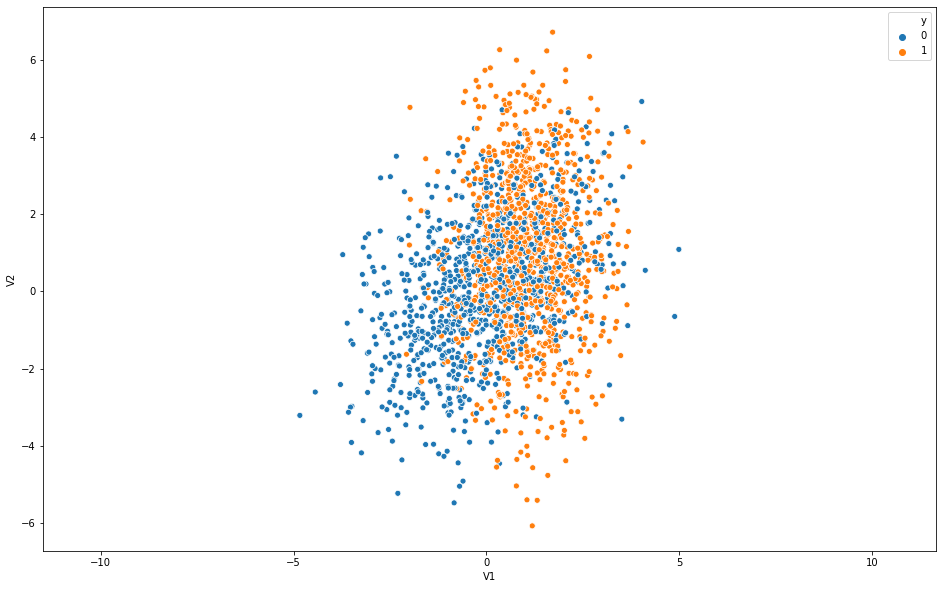In :
# Train a random forest classifier
from sklearn.ensemble import RandomForestClassifier
rf = RandomForestClassifier(n_estimators=100, max_depth=4, random_state=3).fit(train[['V1','V2']], train['y'])
In :
# Select the column with probabilities for class 1 and assign the result to scores variable
scores = pd.DataFrame(rf.predict_proba(val[['V1','V2']]), columns=['y0','y1'])['y1']
In :
from sklearn import metrics
# Using sklearn roc_curve to get thresholds and fpr and tpr
fpr, tpr, thresholds = metrics.roc_curve(val.y, scores, pos_label=1, drop_intermediate=False)
df = pd.DataFrame({'thresholds':thresholds, 'fpr':fpr, 'tpr':tpr})
In :
plt.rcParams['figure.figsize'] = [6, 6]
df.plot(kind='line', x='fpr', y='tpr', title='ROC').set_aspect('equal')
plt.xlabel('fpr')
plt.ylabel('tpr')
plt.show()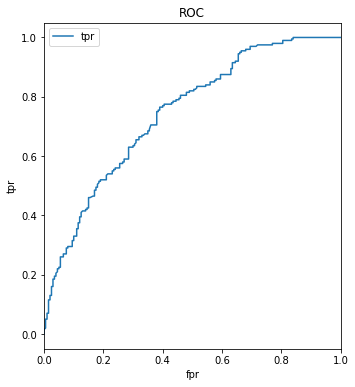Fully Connected Neural Network Model¶

In :
# import libraries
import numpy as np
import pandas as pd
import matplotlib.pyplot as plt

from sklearn.model_selection import train_test_split

from keras.models import Sequential
from keras.layers import Dense, BatchNormalization, Activation, Dropout
In :
num_classes = len(raw_data.target_names)
Out:
mean radius mean texture mean perimeter mean area mean smoothness mean compactness mean concavity mean concave points mean symmetry mean fractal dimension ... worst radius worst texture worst perimeter worst area worst smoothness worst compactness worst concavity worst concave points worst symmetry worst fractal dimension
0 17.99 10.38 122.8 1001.0 0.11840 0.27760 0.3001 0.14710 0.2419 0.07871 ... 25.38 17.33 184.6 2019.0 0.1622 0.6656 0.7119 0.2654 0.4601 0.11890
1 20.57 17.77 132.9 1326.0 0.08474 0.07864 0.0869 0.07017 0.1812 0.05667 ... 24.99 23.41 158.8 1956.0 0.1238 0.1866 0.2416 0.1860 0.2750 0.08902
2 19.69 21.25 130.0 1203.0 0.10960 0.15990 0.1974 0.12790 0.2069 0.05999 ... 23.57 25.53 152.5 1709.0 0.1444 0.4245 0.4504 0.2430 0.3613 0.08758

3 rows × 30 columns

In :
from sklearn.model_selection import train_test_split
X_train, X_test, y_train, y_test = train_test_split(raw_data.data, raw_data.target, train_size=0.7, test_size=0.3, random_state=0)
In :
# Normalize
from sklearn.preprocessing import StandardScaler
scaler = StandardScaler()
scaler.fit(X_train)

X_train = scaler.transform(X_train)
X_test = scaler.transform(X_test)
In :
model = Sequential()

#  The first layer has 8 neurons and expects X_train.shape inputs

model.add(Dense(12, activation ='relu')) # Second layer has 12 nodes

# Add each parameter one by one

model.add(Dense(1, activation ='sigmoid')) # Output is between 1 and 0 using sigmoid function
In :
# Compile model
In :
# Fit the model
model.fit(X_train, y_train, epochs=10, batch_size=100)
Epoch 1/10
398/398 [==============================] - 0s 530us/step - loss: 0.5225 - acc: 0.8744
Epoch 2/10
398/398 [==============================] - 0s 35us/step - loss: 0.4824 - acc: 0.9171
Epoch 3/10
398/398 [==============================] - 0s 37us/step - loss: 0.4448 - acc: 0.9422
Epoch 4/10
398/398 [==============================] - 0s 37us/step - loss: 0.4088 - acc: 0.9447
Epoch 5/10
398/398 [==============================] - 0s 33us/step - loss: 0.3737 - acc: 0.9472
Epoch 6/10
398/398 [==============================] - 0s 32us/step - loss: 0.3394 - acc: 0.9497
Epoch 7/10
398/398 [==============================] - 0s 31us/step - loss: 0.3078 - acc: 0.9523
Epoch 8/10
398/398 [==============================] - 0s 33us/step - loss: 0.2775 - acc: 0.9573
Epoch 9/10
398/398 [==============================] - 0s 40us/step - loss: 0.2497 - acc: 0.9598
Epoch 10/10
398/398 [==============================] - 0s 41us/step - loss: 0.2250 - acc: 0.9623
Out:
<keras.callbacks.History at 0x12d678a90>
In :
# evaluate the model
scores = model.evaluate(X_test, y_test)
print("\n%s: %.2f%%" % (model.metrics_names, scores*100))
171/171 [==============================] - 0s 226us/step

acc: 94.15%